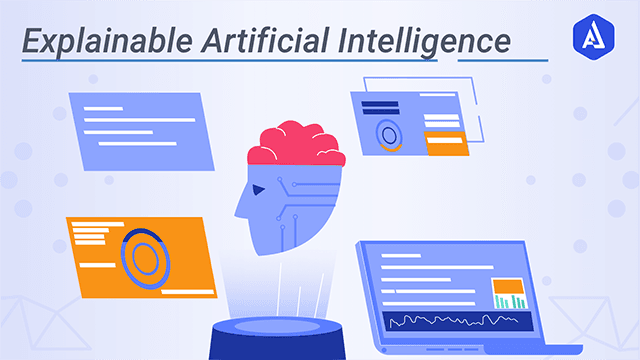top of page
Search

# Stripping iris dataset with 6 explainability Algorithms.

Updated: Jun 5, 2021Explainable AI (XAI) refers to methods and techniques in the application of AI, such that the results of the solution can be understood by human experts. It contrasts with the concept of the 'blackbox in machine learning where even their designers cannot explain why the AI arrived at a specific decision. XAI is an implementation of the social right to explanation.

Here I have taken iris dataset to build a Random Forest. My focus is on providing data explainability, model explainability aka global explainability and prediction aka local explainability.

```## loading data set
from sklearn import datasets
X_df= pd.DataFrame(X, columns=iris.feature_names)
X=iris.data
Y = iris.target```1) Data explainability through IBM AIX 360's Protodash- (https://arxiv.org/abs/1707.01212)-

This algo provides prototypes( samples) of original dataset. So one can see only 10 data points representing entire dataset( million observations) .

Here I want to have only 10 data points as representative of entire dataset.

```
from aix360.algorithms.protodash import ProtodashExplainer, get_Gaussian_Data
explainer = ProtodashExplainer()
(W, S, _) = explainer.explain(X, X, m=10)

# Display the prototypes along with their computed weights
inc_prototypes = X_df.iloc[S, :].copy()
# Compute normalized importance weights for prototypes
inc_prototypes["Weights of Prototypes"] = np.around(W/np.sum(W), 2)

inc_prototypes

```• The data is represented using 10 observations.

• These 10 data points are coming from input data only. See the row index.

• Weights of prototype represents total percentage of similar data points in entire data. Sum of these should be 1.

-----------------------------------------------------------------------------------------------

2) Global Explainability through SHAP's treeexplainer- (https://arxiv.org/pdf/1705.07874.pdf)

SHAP (SHapley Additive exPlanations) is a game theoretic approach to explain the output of any machine learning model. It connects optimal credit allocation with local explanations using the classic Shapley values from game theory and their related extensions

global explanation refers to explaining over-all feature importance in classification.It gives most important variables in model building.

```import shap
explainer = shap.TreeExplainer(model, data=X_df)

shap.summary_plot(shap_values, X-df, plot_type="bar")```• colors in the bar represents relative contribution in classifying three classes.

• Over all petal length and width are more important that sepal length and width.

• This is kind of variable importance we get many many algos.

-----------------------------------------------------------------------------------------------

3) Global explainability through IBM360 LRR ( Logistic rule regression )- ( Wei et al., 2019)

```Logistic Rule Regression is a directly interpretable supervised learning
method that performs logistic regression on rule-based features```

```# Generalized Linear Rule Models
from aix360.algorithms.rbm import FeatureBinarizer
from aix360.algorithms.rbm import LogisticRuleRegression
from sklearn.metrics import accuracy_score
fb = FeatureBinarizer(negations=True, returnOrd=True)
dfTrain, dfTrainStd = fb.fit_transform(X_df)

lrr = LogisticRuleRegression(lambda0=0.005, lambda1=0.001, useOrd=True, maxSolverIter=10000)
lrr.fit(dfTrain, Y, dfTrainStd)
print('Training accuracy:', accuracy_score(Y, lrr.predict(dfTrain, dfTrainStd)))
print('where z is a linear combination of the following rules/numerical features:')
lrr.fit(dfTrain, y, dfTrainStd)

lrr.explain()

```• so LRR builds a surrogate model and provides importance of rules created from the features.

• This is important as one can see overall importance of a variable but even the over-all importance may vary for qualities/range of the same variable.

• In the above example sepal width <=3 is better classifier than sepal width <=3.2.-----------------------------------------------------------------------------------------------

4) Local explanation through LIME Tabular- https://arxiv.org/pdf/1602.04938.pdf

As we see in LRR, a variable may be important in classifying most of the instances but may not be important for all the instances. ( instance=data point).

To see the feature importance of a particular prediction, we should look for local explainability.

There is a lot of work already happened.I am presenting a few-

```import lime
explainer = lime.lime_tabular.LimeTabularExplainer(X,
feature_names=X_df.columns, class_names=iris.target_names, discretize_continuous=True)

exp = explainer.explain_instance(X_df.iloc[1,:], model.predict_proba, num_features=10, top_labels=1)

exp.show_in_notebook(show_table=True, show_all=False)```• Predicted probabilities are output class probabilities.

• Horizontal bar plot shows features contributing to output class which is setosa in above example. Coffecient .49, .41 represents relative importance of features,

• Values of feature for that instance is also given in 3rd table.[ feature-value table]

• Explanation is for a datapoint- X_df.iloc[1,:].

-----------------------------------------------------------------------------------------------

5) Local explanation through treeinterpreter- (https://pypi.org/project/treeinterpreter/)

TreeInterpreter decomposes the predictions into the bias term (which is just the trainset mean) and individual feature contributions, so one can see which features contributed to the difference and by how much.

[line 2 in below code]

```from treeinterpreter import treeinterpreter as ti
prediction, bias, contributions = ti.predict(model, X_test)
# converting 3 d to 2 d, 1 instance at a time-
contributions= contributions
pd_contribution= pd.DataFrame(contributions)

pd_contribution.columns= iris.target_names
pd_contribution.index= iris.feature_names
pd_contribution['Overall Importance']=abs(pd_contribution['setosa'])+abs(pd_contribution['versicolor'])+abs(pd_contribution['virginica'])
pd_contribution.sort_values('Overall Importance', ascending=False, inplace=True)

print(pd_contribution)```• table shows the importance of features in classifying all the classes for a datapoint(first data point here as I have taken contributions= contributions. )

• Overall importance shows how well a feature is doing in classifying all the classes.

-----------------------------------------------------------------------------------------------

6) Local explanation through SHAP Kernal Explainer-

SHAP has 7 different explainability algos. Kernal Shap is one of them. It uses a specially-weighted local linear regression to estimate SHAP values for any model. So it is model agnostic also.[ works for any blackbox model]

```import shap
x_test_instance= X[149,:]
shap_values = explainer.shap_values(X, nsamples=100)
shap_values[149,:]
shap.force_plot(explainer.expected_value, shap_values[149,:], x_test_instance, iris.feature_names,• The above explanation shows three features each contributing to push the model output from the base value(.333) (the average model output over the training dataset we passed) towards zero.

• Features pushing class label higher are shown in red.

-----------------------------------------------------------------------------------------------

There are many frameworks available for explainability. like-

Aix360 Alibi Dalex Eli5

H20 Google explainable AI Skater Lucid- tensorflow

Captum MS Azure explainability InterpretML LIME/SHAP

do try these and kill the dataset next time.

another article on what to include from all the above crap in any ML model-

```http://machinelearningstories.blogspot.com/2019/12/explainability-in-data-science-data.html

```